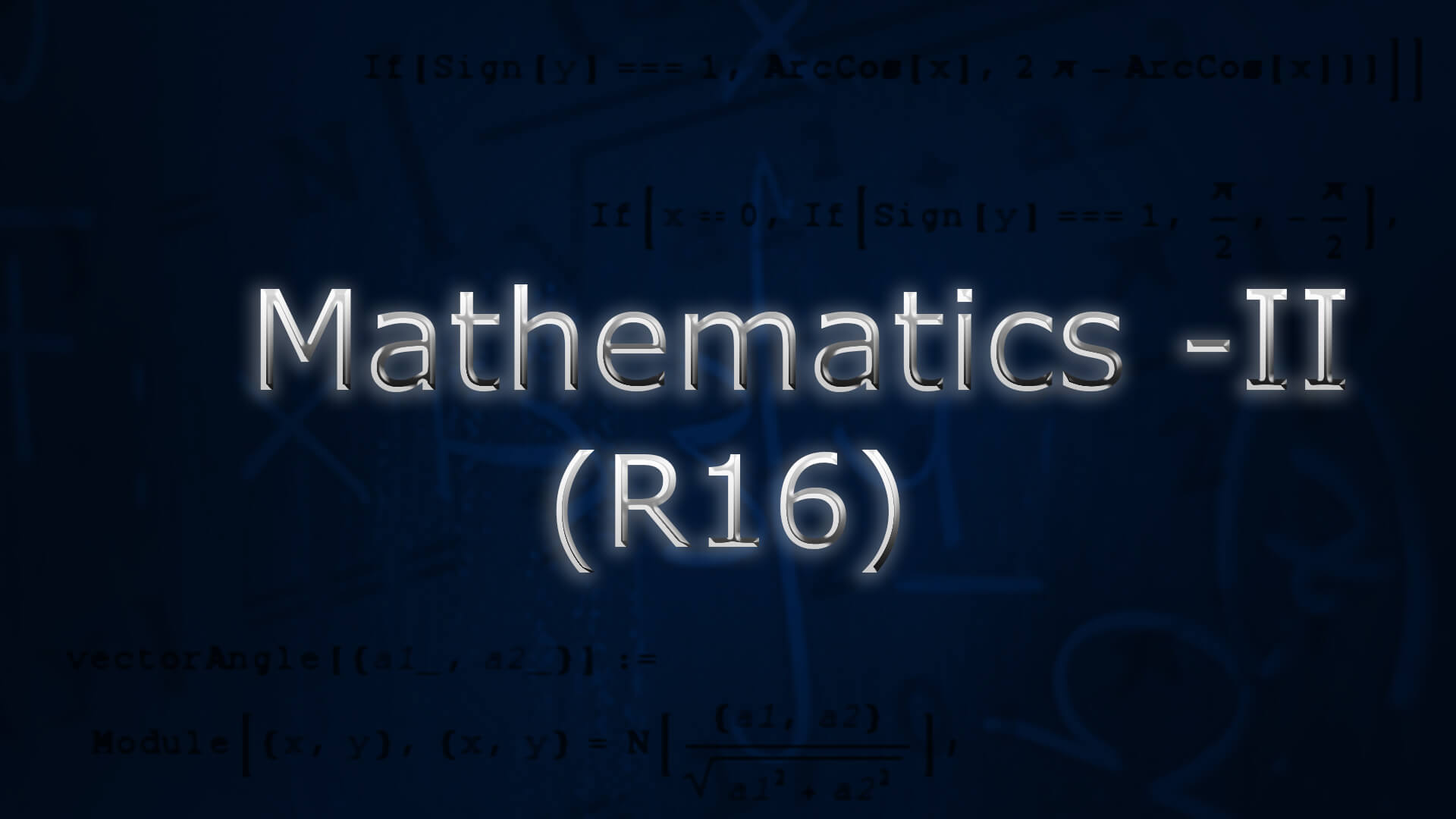## Mathematics-II (R16) by Dr C N B Rao ., M.Sc ,Ph.D (IIT Kharagpur ) The course is designed to equip the students with the necessary mathematical skills and techniques that are essential for an engineering course. The skills derived from the course will help the student from a necessary base to develop analytic and design concepts. Code : M2R16B.Tech Recommended For : B.Tech 1st Year JNTUH

#### After Learning this subject,you should be able to

• Use Laplace transform techniques for solving DE’s.
• Evaluate integrals using Beta and Gamma functions
• Evaluate the multiple integrals and can apply these concepts to find areas, volumes, moment of inertia etc of regions on a plane or in space
• Evaluate the line, surface and volume integrals and converting them from one to another.

## Curriculum 08:21:30   units   |   6

• 2:10:31

UNIT 1
Laplace Transforms: Introduction, transforms of elementary functions, transforms of elementary functions-PART 01, transforms of elementary functions Problems, transforms of elementary functions Problems Part 1, First Shifting theorem, Problems on First Shifting theorem, Properties of laplace transforms, properties of laplace transforms Part 1, Transform of Derivatives & Integrals, Problems transforms of derivatives, transforms of integrals, Multiplication by t power n& Division by t, multiplication by t power n, division by t .(statements only), Dirac�s delta function, Periodic�function

• 1:25:48

UNIT 1.2
Inverse transforms: IT Introduction, Basic Formulas of Inverse Laplace Transform, Problems on Inverse Laplace Transform, Method of Partial fraction, Method of Partial fraction Part 1, First Shifting theorem (Table), Problem on First Shifting theorem, Second Shifting Theorem, Inverse Laplace Transform of Derivatives,Integrals, Multiplication & Division by S, IT Convolution theorem, Convolution theorem (without proof), applications to differential equations., applications to differential equations - Part 1

• 1:04:12

UNIT 2
Beta and Gamma Functions: Gamma Function, Gamma Function Part 1, Beta Function, Properties, Relation between Beta and Gamma functions, Relation between Beta and Gamma functions - Part 1, Relation between Beta and Gamma functions - Part 2, Applications to evaluation of improper integrals, The error function & Complimentory error function, Evaluation of integrals using Beta and Gamma functions

• 1:40:55

UNIT 3
Multiple Integrals: Introduction, Double Integrals, Triple Integrals, Triple Integrals Part 1, Change of variables, Change of order of integration, Applications to find Areas, Applications to find Areas Part 1, Moment of Inertia and volumes, Moment of Inertia and volumes Part 1, volumes & Center of gravity (evaluation using Beta�and Gamma functions)

• 1:58:44

UNIT 4
Vector Differentiation: Introduction to Vector Differentiation, Gradient, Directional derivative, Problems on Gradient and Directional Derivative - Part 1, Problems on Gradient and Directional Derivative - Part 2, Divergence, Curl, Problems on Gradient,Divergence and Curl, Problems on Gradient,Divergence and Curl Part 1, Incompressible flow, Solenoidal and irrotational vector fields, Second order Operators, Del applied twice to point function, Del applied twice to point function Problems, Del applied twice to point function Problems Part 1, Del applied to products of point functions, Problems on Del applied to products of point functions, Problems on Del applied to products of point functions Part 1, �Laplacian operator, Vector identities

• 1:27:08

UNIT 5
Vector Integration: Line Integral, Problems On Work Done, Problems On Work Done - Part 1, Potential Function, Area,surface and volume of integrals, Flux, Vector integral theorems, Greens Theorem, Green's Theorem Problems, Stokes Theorem, Stokes Theorem Part 1, Stokes Theorem Part 2, Gauss Divergence theorem, Gauss Divergence theorem Part 1, Problems